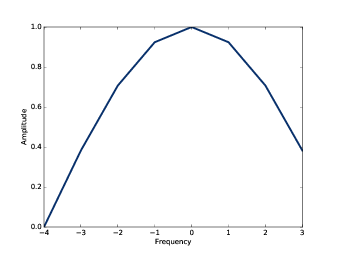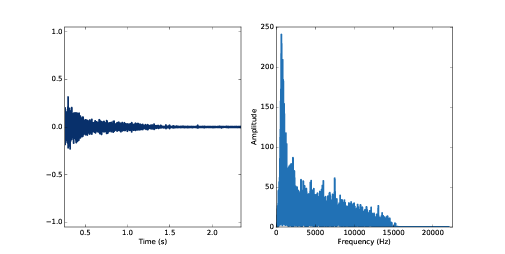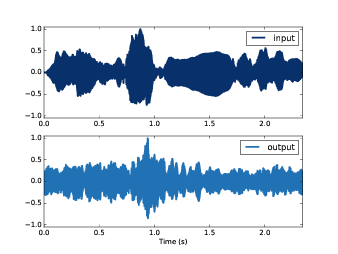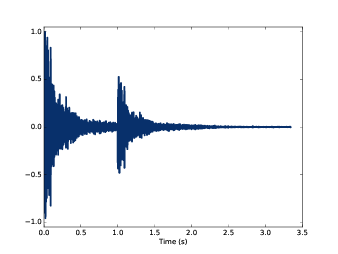# 第十章：线性时不变系统¶

## 10.1 信号与系统¶

1. 线性：如果你同时输入两个不同的信号，那么得到的输出和这两个信号各自的输出之和相同。 用数学语言描述为，如果输入 x1 产生输出 y1 ，输入 x2 产生输出 y2，那么 输入 ax1+bx2 产生的输出为 ay1+by2 。这里 ab 为常数。
2. 时不变性：系统的作用效果不随时间的变化而变化，仅依赖于系统本身的状态。因此，如果输入变化仅是在时间上平移， 那么产生的输出变化也仅仅是相同的时间平移，其他都是一样的。

• 仅包含电阻，电容和电感的电路系统是LTI。这里我们只把这些元件考虑为理想模型。
• 包含弹簧，块和阻尼装置的机械系统同样是LTI。这里假定弹簧和阻尼器是线性的（力与位移成正比，力与速度成正比）
• 本书中的讲的最多的声音的传输介质（包括空气，水和固体等）也可以使用LTI作为近似模型。

LTI可以由线性微信方程描述，而它的解可以用复指数函数来表示， 见 http://en.wikipedia.org/wiki/Linear_differential_equation

1. 将输入信号表示为不同频率的复指数函数之和。
2. 对每个分量计算相应的输出
3. 将所有的输出加起来就得到了最终的输出

impulse = np.zeros(8)
impulse = 1
impulse_spectrum = np.fft.fft(impulse)


[ 1.  0.  0.  0.  0.  0.  0.  0.]


[ 1.+0.j  1.+0.j  1.+0.j  1.+0.j  1.+0.j  1.+0.j  1.+0.j  1.+0.j]


## 10.2 窗和滤波器¶

window_array = np.array([0.5, 0.5, 0, 0, 0, 0, 0, 0,])
window = thinkdsp.Wave(window_array, framerate=8)
filtr = window.make_spectrum(full=True)product = impulse_spectrum * filtr
filtered = product.make_wave()


• 由于脉冲信号的频谱都是1，因此脉冲响应的DFT与系统的滤波响应是相同的。
• 脉冲响应与描述系统的卷积窗也是相同的。

## 10.3 声学响应¶

response = thinkdsp.read_wave('180961__kleeb__gunshots.wav')
response = response.segment(start=0.26, duration=5.0)
response.normalize()
response.plot()transfer = response.make_spectrum()
transfer.plot()


violin = thinkdsp.read_wave('92002__jcveliz__violin-origional.wav')
violin.truncate(len(response))
violin.normalize()


spectrum = violin.make_spectrum()


output = (spectrum * transfer).make_wave()
output.normalize()
output.plot()## 10.4 系统和卷积¶

• 脉冲信号是有幅值均为1的所有频率组成的
• 脉冲响应是系统对所有频率的响应之和
• 脉冲响应的DFT，即传递函数，它表征了系统对所有频率的响应。
• 任何信号经过系统的输出，均可以表示为输入信号的所有频率成分经过系统后响应之和。

• 输入信号的采样值可以表示为一系列幅值不同时移不同的脉冲信号
• 由于系统是时不变的，每个脉冲信号都会产生相应的不同幅值不同时移的脉冲响应
• 由于系统是线性的，系统的输出应该等于这些不同的脉冲响应之和

def shifted_scaled(wave, shift, factor):
res = wave.copy()
res.shift(shift)
res.scale(factor)
return res


shift = 1
factor = 0.5
gun2 = response + shifted_scaled(response, shift, factor)dt = 1 / 441
total = 0
for k in range(100):
total += shifted_scaled(response, k*dt, 1.0)


signal = thinkdsp.SawtoothSignal(freq=441)
wave = signal.make_wave(duration=0.1,
framerate=response.framerate)


total = 0
for t, y in zip(wave.ts, wave.ys):
total += shifted_scaled(response, t, y)$h = fg + fg + fg$

h[n] = sumlimits_{m = 0}^{N - 1} {f[m]g[n - m]}

8.2 我们就见过这个式子了，它就是 fg 的卷积。 说明如果系统的脉冲响应为 g ，那么系统输入 f 产生的输出应该为 fg 的卷积。

1. 把输入理解为一系列的脉冲信号，那么输出就是脉冲响应进行时移和缩放后的和， 也就是输入与脉冲响应的卷积。
2. 脉冲响应的DFT是系统的传递函数，它包含了系统对每个频率成分的影响效果，而输入可以理解为 不同频率的分量之和，因此将输入的DFT乘以传递函数就可以得到输出的DFT。

## 10.5 卷积定理的证明¶

$DFT(f*g) = DFT(f)DFT(g)$

1. 我会先从 f 为复指数信号这个特例开始，说明它与 g 的卷积相当于对 f 乘以一个标量
2. 然后我们把 f 推广到一般的信号，它可以表示为不同频率的复指数信号之和，然后通过乘以一个标量 来计算各个频率成分的卷积，再把结果加起来

$DFT(g)[k] = G[k] = \sum\limits_n {g(n){e^{ - 2\pi ink/N}}}$

f 的IDFT 写作 Ff 等于：

$IDFT(F)[n] = f[n] = \sum\limits_k {F[k]{e^{2\pi ink/N}}}$

$(f*g)[n] = \sum\limits_m {f[m]g[n - m]}$

$(f*g)[n] = \sum\limits_m {f[n - m]g[m]}$

$f[n] = {e_k}[n] = {e^{2\pi ink/N}}$

$({e_k}*g)[n] = \sum\limits_m {{e^{2\pi i(n - m)k/N}}g[m]}$

$({e_k}*g)[n] = {e^{2\pi ink/N}}\sum\limits_m {{e^{ - 2\pi imk/N}}g[m]}$

$({e_k}*g)[n] = {e_k}[n]G[k]$

$(f*g)[n] = \sum\limits_k {F[k]G[k]{e_k}[n]}$

$(f*g)[n] = \sum\limits_k {F[k]G[k]{e^{2\pi ink/N}}}$

$f*g = IDFT(FG)$

$f*g = IDFT(DFT(f)DFT(g))$

$DFT(f*g) = DFT(f)DFT(g)$

## 10.6 练习¶

chap10.ipynb 中的例子进行补0后再计算，以消除这个问题对结果的影响。